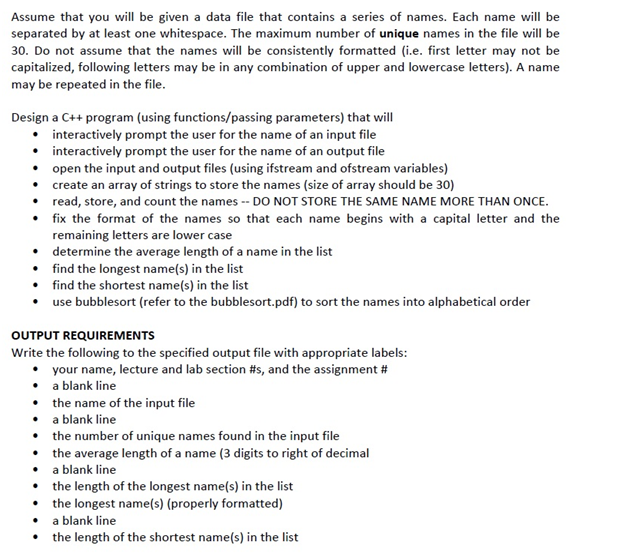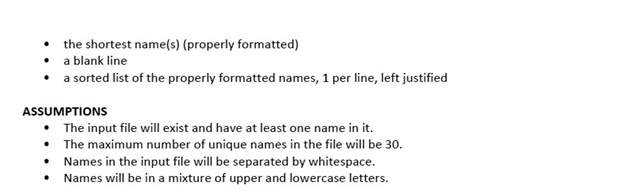# How to sort and format names in a list

Check out the following assignment sample where our experts give a detailed explanation on how to format and sort a list of names. If you are struggling with an assignment of this nature, feel free to hire them to do the task for you.

## Sort and Format a List of Names#include

#include

#include

#include

#include

using namespace std;

constint MAX_SIZE = 30; //maximum number of unique names in data file

//Functions to be used

voidfixname(string&);

void unique(string[], string[], string)

voidbubblesort(string secondArray[], int count)

int main()

{

ifstreaminfile; //variable representing input file

ofstreamoutfile; //variable representing output file

stringinputf,outputf; //name of input file and output file

string name; //name read from input file

stringfirstArray[MAX_SIZE]; //array of names from data file

stringsecondArray[MAX_SIZE]; //second array to store unique names in

cout<< “enter input file name” <

cin>>inputf;

infile.open(inputf.c_str());

if (!infile)

{

return 0;

}

else

{

cout<< “enter output file name” <

cin>>outputf;

outfile.open(outputf.c_str(),ios::app);

infile>> name;

for (int i = 0; i < MAX_SIZE; i++)

{

infile>>firstArray[i];

}

}

while (infile)

{

}

infile.close();

}

return 0;

}

voidbubblesort(string secondArray[], int count) //This function will use the bubblesort method to sort the list of \

names in alphabetical (ascending) order.

{

string temp;

for (int i = 0; i < count – 1; i++)

for (int j = 0; j < count – (i + 1); j++)

if (secondArray[j] >secondArray[j+1])

{

temp = secondArray[j];

secondArray[j] = secondArray[j+1];

secondArray[j+1] = temp;

}

}

void unique(string firstArray[], string secondArray[], string name) //This function will compare all of the strings \

from the data file and keep a count of unique strings found. It will also store the unique names in the second array\

{

int n = 0;

for (int i = 0; i < count; i++) //this needs to use count and not the constant for MAX_SIZE

for (int j = 0; j < i; j++)

if ((j != i) && (firstArray[j] != firstArray[i]))

{

secondArray[n] = firstArray[i];

count++;

n++;

}

return;

}

voidfixname(string& name) //This function will properly format the names in the output file.

{

int n; //length of name

n = name.length();

name = toupper(name); //This will change the first character of each name to a capital letter

for (int i = 1; i < n; i++)

name[i] = tolower(name[i]); //This will change the remaining letters to all lowercase.

}

//Function for finding length of longest name(s)

//Function for finding length of shortest name(s)

//Function for printing results to output file

Solution

#include

#include

#include

#include

#include

using namespace std;

constint MAX_SIZE = 30; //maximum number of unique names in data file

//Functions to be used

voidfixname(string&);

voidbubblesort(string[], int);

int max(int[],int);

int min(int[],int);

voidout_write(string, string, int, double, int, int, int[], string[]);

int main()

{

ifstreaminfile; //variable representing input file

stringinputf,outputf; //name of input file and output file

string name; //name read from input file

stringfirstArray[MAX_SIZE]; //array of names from data file

int length[MAX_SIZE]; //second array to store unique names in

int flag, size, count=0,sum=0;

doubleavg_length;

intmax_length, min_length;

cout<< “enter input file name” <

cin>>inputf;

infile.open(inputf.c_str());

if (!infile)

{

return 0;

}

else

{

cout<< “enter output file name” <

cin>>outputf;

for (int i = 0; i

{

infile>> name;

fixname(name);

flag = 1;

for (int j = 0;j

if (name == firstArray[j]){ //checking uniqueness

flag = -1;

break;

}

}

if (flag == 1){

firstArray[count] = name;

length[count] = name.length();

sum = sum + length[count];

count++;

}

}

avg_length = (1.0*sum)/count;

max_length = max(length,count);

min_length = min(length,count);

}

infile.close();

out_write(inputf,outputf,count,avg_length,max_length,min_length,length,firstArray);

return 0;

}

voidbubblesort(string firstArray[], int count) //This function will use the bubblesort method to sort the list of \

names in alphabetical (ascending) order.

{

string temp;

for (int i = 0; i < count – 1; i++)

for (int j = 0; j < count – (i + 1); j++)

if (firstArray[j] >firstArray[j+1])

{

temp = firstArray[j];

firstArray[j] = firstArray[j+1];

firstArray[j+1] = temp;

}

}

voidfixname(string& name) //This function will properly format the names in the output file.

{

int n; //length of name

n = name.length();

name = toupper(name); //This will change the first character of each name to a capital letter

for (int i = 1; i < n; i++)

name[i] = tolower(name[i]); //This will change the remaining letters to all lowercase.

}

//Function for finding length of longest name(s)

int max(int length[], int count){

intmax_length = length;

for (int i = 0;i

if (length[i]>max_length)

max_length = length[i];

}

returnmax_length;

}

//Function for finding length of shortest name(s)

int min(int length[], int count){

intmin_length = length;

for (int i = 0;i

if (length[i]

min_length = length[i];

}

returnmin_length;

}

//Function for printing results to output file

voidout_write(string inputf, string outputf, int count, double avg_length, intmax_length, intmin_length, int length[], string firstArray[]){

ofstreamoutfile; //variable representing output file

outfile.open(outputf.c_str());

outfile<< “name section lab_section assignment\n\n”;

outfile<< “Input file: ” <

outfile<< “Number of unique names: ” << count <<“\n”;

outfile<< “Average length of a name: ” <

outfile<< “Length of longest names: ” <

outfile<< “Longest names: “;

for (int i = 0;i

if (length[i]==max_length)

outfile<

}

outfile<< “\n\nLength of longest names: ” <

outfile<< “Shortest names: “;

for (int i = 0;i

if (length[i]==min_length)

outfile<

}

outfile<

bubblesort(firstArray,count);

for (int i = 0; i < count; i++)

{

outfile<< “\n” <

}

outfile.close();

}